# How to compare and match between 2 excels with similar data?

Member Posts: 3Newbie
I have 2 excels. Both have the company name and country data. But the company names are similar and are not the same. So using the country data (which is the same), I have to match the company names and display the final matched data in one excel file. I have also attached the example of the data in both excels. I have colour coded it so that they can be understood as the similar company names (Cat INC = CAT LLP). I created a model which uses operators like replace (with lots of manual work like entering the replaceable values). Also, the real data file consists of 1000's of rows in it. So it would be helpful if someone could suggest a model type which can compare and match data between 2 files.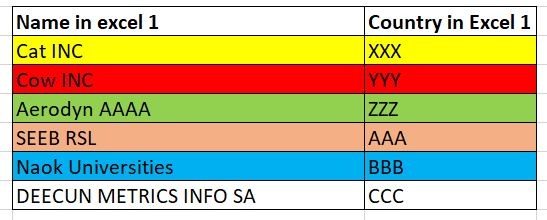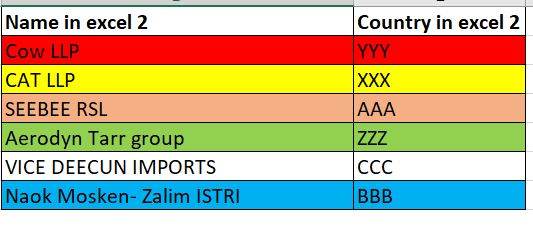• Member Posts: 662Unicorn
You can use the join operators for this, in combination with the set minus one. First you link the excel files so you get 2 datasets, you give your name the ID role, inner join the 2 datasets, as these will be the common ones, and link this back with your original set using the minus operator. This will then tell you what was missing (or different in this case).
• Administrator, Employee, RapidMiner Certified Analyst, RapidMiner Certified Expert, Member Posts: 363RM Data Scientist
Hi @Jayanthan12,

Do you have toolbox extension installed to try the new "fuzzy matching" operator? It will use the popular Levenshtein distance or any other variation distance measures to merge two tables with fuzzy matching. It will show several number of candidate matches as you want.
You can apply a filter right after the fuzzy matching to make sure the county names are exactly the same.

Sample process is here
```<?xml version="1.0" encoding="UTF-8"?><process version="9.8.001">
<context>
<input/>
<output/>
<macros/>
</context>
<operator activated="true" class="process" compatibility="9.8.001" expanded="true" name="Process">
<parameter key="logverbosity" value="init"/>
<parameter key="random_seed" value="2001"/>
<parameter key="send_mail" value="never"/>
<parameter key="process_duration_for_mail" value="1"/>
<parameter key="encoding" value="SYSTEM"/>
<process expanded="true">
<operator activated="true" class="utility:create_exampleset" compatibility="9.8.001" expanded="true" height="68" name="Create ExampleSet" width="90" x="45" y="85">
<parameter key="generator_type" value="comma separated text"/>
<parameter key="number_of_examples" value="100"/>
<parameter key="use_stepsize" value="false"/>
<list key="function_descriptions"/>
<list key="numeric_series_configuration"/>
<list key="date_series_configuration"/>
<list key="date_series_configuration (interval)"/>
<parameter key="date_format" value="yyyy-MM-dd HH:mm:ss"/>
<parameter key="time_zone" value="SYSTEM"/>
<parameter key="input_csv_text" value="Name1,Country1&#10;Cat INC,XXX&#10;Cow INC,YYY&#10;Aerodyn AAAA,ZZZ&#10;SEEB RSL,AAA&#10;Naok Universities,BBB&#10;DEECUN METRICS INFO SA,CCC"/>
<parameter key="column_separator" value=","/>
<parameter key="parse_all_as_nominal" value="false"/>
<parameter key="decimal_point_character" value="."/>
<parameter key="trim_attribute_names" value="true"/>
</operator>
<operator activated="true" class="utility:create_exampleset" compatibility="9.8.001" expanded="true" height="68" name="Create ExampleSet (2)" width="90" x="45" y="238">
<parameter key="generator_type" value="comma separated text"/>
<parameter key="number_of_examples" value="100"/>
<parameter key="use_stepsize" value="false"/>
<list key="function_descriptions"/>
<list key="numeric_series_configuration"/>
<list key="date_series_configuration"/>
<list key="date_series_configuration (interval)"/>
<parameter key="date_format" value="yyyy-MM-dd HH:mm:ss"/>
<parameter key="time_zone" value="SYSTEM"/>
<parameter key="input_csv_text" value="Name2,Country2&#10;Cow LLP,YYY&#10;CAT LLP,XXX&#10;SEEBEE RSL,AAA&#10;Aerodyn Tarr group,ZZZ&#10;VICE DEECUN IMPORTS,CCC&#10;Naok Mosken- Zalim ISTRI,BBB"/>
<parameter key="column_separator" value=","/>
<parameter key="parse_all_as_nominal" value="false"/>
<parameter key="decimal_point_character" value="."/>
<parameter key="trim_attribute_names" value="true"/>
</operator>
<operator activated="true" class="operator_toolbox:fuzzy_matching" compatibility="2.8.001" expanded="true" height="82" name="Fuzzy Matching" width="90" x="447" y="85">
<parameter key="left_side_attribute" value="Name1"/>
<parameter key="right_side_attribute" value="Name2"/>
<parameter key="number_of_matches" value="3"/>
<parameter key="similarity_measure" value="LEVENSHTEIN_TOKEN_SORT_RATIO"/>
<description align="center" color="transparent" colored="false" width="126">using Levenshtein ratio or any variations to define the similarity measurement for fuzzy matching of two names</description>
</operator>
<operator activated="true" class="filter_examples" compatibility="9.8.001" expanded="true" height="103" name="Filter Examples" width="90" x="648" y="85">
<parameter key="parameter_expression" value="Country1==Country2"/>
<parameter key="condition_class" value="expression"/>
<parameter key="invert_filter" value="false"/>
<list key="filters_list"/>
<parameter key="filters_logic_and" value="true"/>
</operator>
<connect from_op="Create ExampleSet" from_port="output" to_op="Fuzzy Matching" to_port="left"/>
<connect from_op="Create ExampleSet (2)" from_port="output" to_op="Fuzzy Matching" to_port="right"/>
<connect from_op="Fuzzy Matching" from_port="matched" to_op="Filter Examples" to_port="example set input"/>
<connect from_op="Filter Examples" from_port="example set output" to_port="result 1"/>
<portSpacing port="source_input 1" spacing="0"/>
<portSpacing port="sink_result 1" spacing="0"/>
<portSpacing port="sink_result 2" spacing="0"/>
</process>
</operator>
</process>```

Cheers,
YY
• Member Posts: 3Newbie
I want to give a clarification on my query.

I have 2 excels. Both have the company and country name. But the company names are similar and are not the same. I have to match the company names(even if one of the words in the names are matching, eg:Cat INC and CAT LLP should be matched) and display the final matched data in one excel file as shown below (3). I have also attached the example of the data of both excels (1&2). I have colour coded it so that they can be understood as the similar company names (Cat INC = CAT LLP).  Also, the real data file consists of 1000's of rows in it. So it would be helpful if someone could suggest a model type which can compare and match data between 2 files.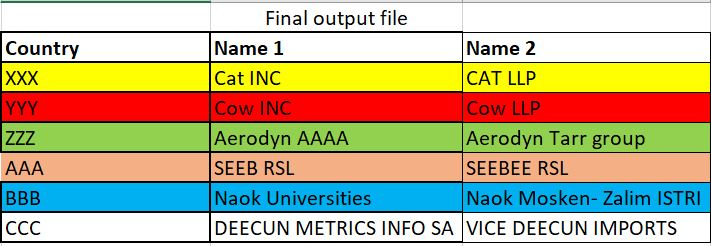• Administrator, Employee, RapidMiner Certified Analyst, RapidMiner Certified Expert, Member Posts: 363RM Data Scientist
The fuzzy matching and filter can be used to fuzzy join these two input tables
You can load data from "Read Excel" and give it a try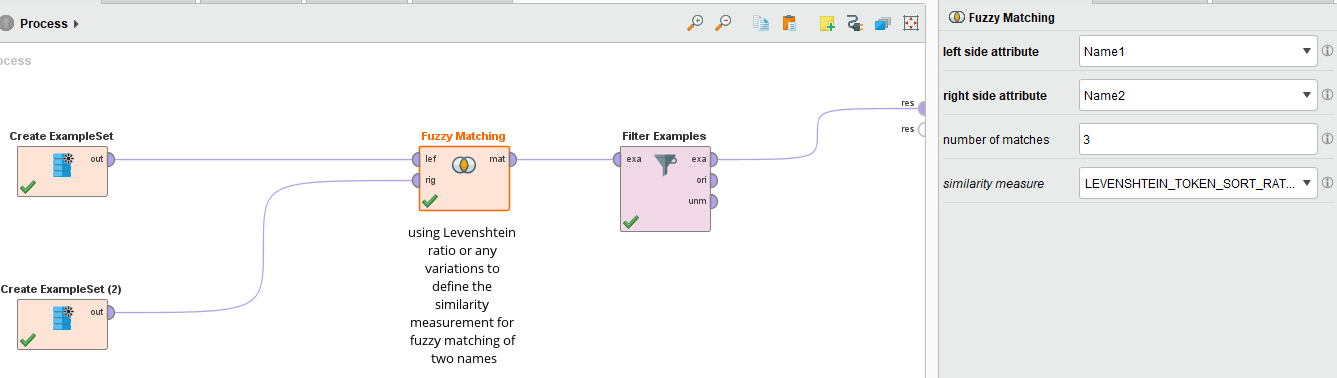Output is like this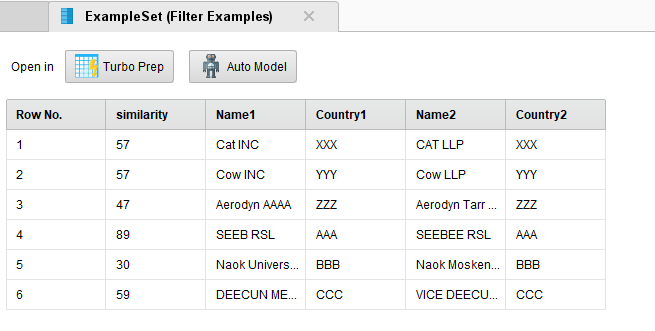HTH!

YY